# Thresholding#

Thresholding is a technique of image segmentation. It separates a given single-channel image (or stack) into two regions: Pixels with intensity below a given threshold, also called “background” and pixels with intensity above a given threshold, “foreground”. Typically those algorithms result in binary images where background intensity is 0 and foreground intensity is 1. When applying such algorithms in ImageJ, foreground pixels are 255. In scikit-image, background pixels are False and foreground pixels are True.

from skimage.io import imread
from pyclesperanto_prototype import imshow
import pyclesperanto_prototype as cle

from skimage import filters
from skimage.filters import try_all_threshold
from matplotlib import pyplot as plt
import napari_simpleitk_image_processing as nsitk

image = imread("../../data/blobs.tif")
imshow(image)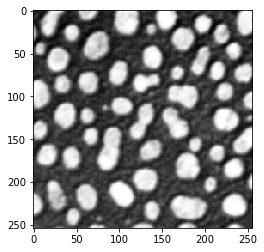## Image segmentation by thresholding#

The threshold_otsu operation, also known as Otsu’s method (Otsu et al., IEEE Transactions on Systems, Man, and Cybernetics, Vol. 9 (1), 1979), delivers a number - the threshold to be applied.

threshold = filters.threshold_otsu(image)


When using methods such as thresholding in notebooks, it is recommended to print out the result to see what it actually returns. Here, we are using the method from scikit-image, which returns the threshold that is applied. Printing that threshold can be helpful later when reproducing the workflow, also if others want to apply the same threshold to the dataset in other software.

threshold

120


Using numpy arrays, we can apply the threshold by applying the >= operator. The result will be a binary image.

binary_image = image >= threshold

imshow(binary_image)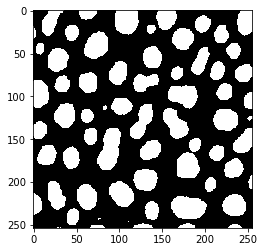We can also determine in which type the binary image is processed by printing out minimum and maximum of the image:

binary_image.max()

True

binary_image.min()

False


As shown earlier, matplotlib allows us to draw an outline on top of an image visualized using imshow using the contour command.

# create a new plot
fig, axes = plt.subplots(1,1)

axes.imshow(image, cmap=plt.cm.gray)
axes.contour(binary_image, [0.5], linewidths=1.2, colors='r')

<matplotlib.contour.QuadContourSet at 0x2b57076dc70>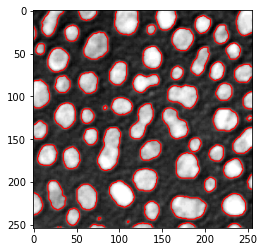There is a list of thresholding algorithms available. It is possible to apply them all to your data and see differences:

fig, ax = try_all_threshold(image, figsize=(10, 8), verbose=False)
plt.show()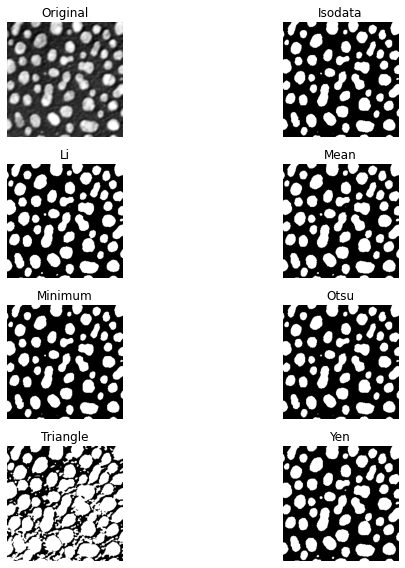## Thresholding using pyclesperanto#

Furthermore, also other libraries such as pyclesperanto offer thresholding algorithms. The implementation here does not return the threshold, it directly returns the binary image.

binary_image2 = cle.threshold_otsu(image)
imshow(binary_image2)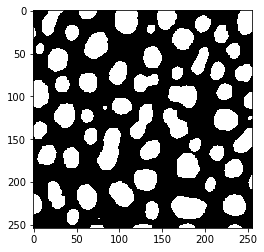Here we can also see that different libraries store binary images in different ways. pyclesperanto for example stores the positive pixels in binary images not as True but with a 1 instead:

binary_image2.max()

1.0

binary_image2.min()

0.0


## Thresholding using SimpleITK#

Also SimpleITK offers thresholding algorithms which can be found in the list of filters. For scripting convenience, we use here napari-simpleitk-image-processing a scriptable napari plugin that offers some SimpleITK functions in a more accessible way. We can program a small for-loop that tries all the thresholding alogrithms in SimpleITK and shows us the results:

threshold_algorithms = [
nsitk.threshold_huang,
nsitk.threshold_intermodes,
nsitk.threshold_isodata,
nsitk.threshold_kittler_illingworth,
nsitk.threshold_li,
nsitk.threshold_maximum_entropy,
nsitk.threshold_moments,
nsitk.threshold_otsu,
nsitk.threshold_renyi_entropy,
nsitk.threshold_shanbhag,
nsitk.threshold_triangle,
nsitk.threshold_yen
]

for algorithm in threshold_algorithms:
# show name of algorithm above the image
print(algorithm.__name__)

# binarize the image using the given algorithm
binary_image = algorithm(image)

# show the segmentation result
imshow(binary_image)

threshold_huang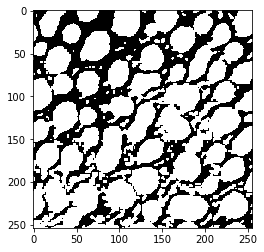threshold_intermodes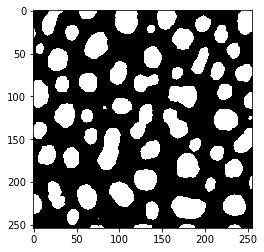threshold_isodatathreshold_kittler_illingworth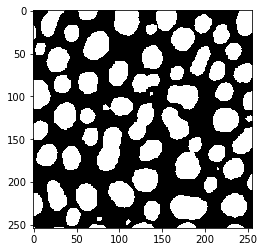threshold_li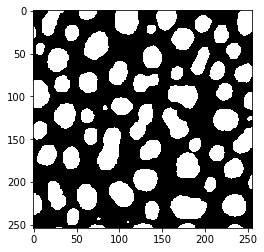threshold_maximum_entropythreshold_momentsthreshold_otsuthreshold_renyi_entropy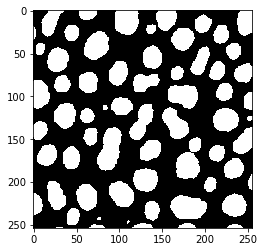threshold_shanbhag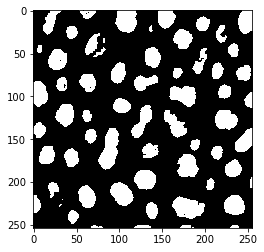threshold_trianglethreshold_yen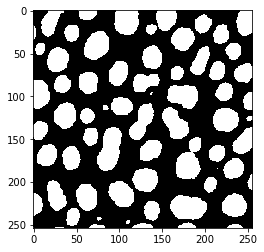# Exercise#

Segment blobs.tif using the Yen algorithm. Use matplotlib to draw a green outline of the segmented objects around the regions on the original image.

Segment the image using a calculated threshold according to this equation:

threshold = mean + 2 * standard_deviation


Visualize the resulting segmentation with a red outline on top of the original image and the green outline from above.

Alternatively, put both segmentation results in napari and compare it there visually.